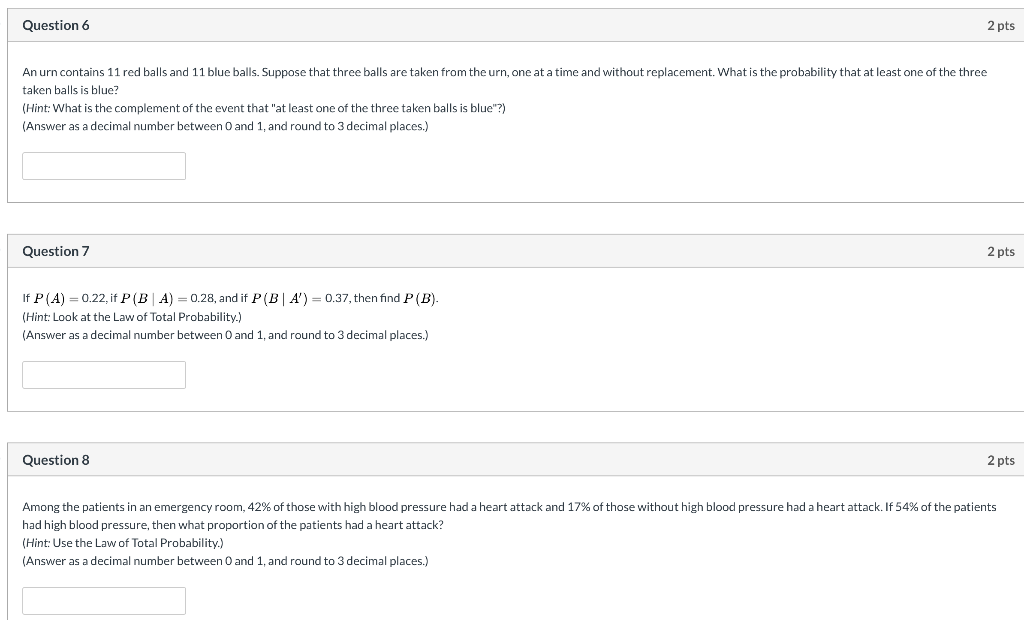Home / Answered Questions / Other / question-6-2-pts-an-urn-contains-11-red-balls-and-11-blue-balls-suppose-that-three-balls-are-taken-f-aw871

# (Solved): Question 6 2 Pts An Urn Contains 11 Red Balls And 11 Blue Balls. Suppose That Three Balls Are Taken ...Question 6 2 pts An urn contains 11 red balls and 11 blue balls. Suppose that three balls are taken from the urn, one at a time and without replacement. What is the probability that at least one of the three taken balls is blue? (Hint: What is the complement of the event that "at least one of the three taken balls is blue"?) (Answer as a decimal number between 0 and 1, and round to 3 decimal places.) Question 7 2 pts If P(A) = 0.22, if P(B A) = 0.28, and if P ( B A ) = 0.37, then find P(B). (Hint: Look at the Law of Total Probability.) (Answer as a decimal number between 0 and 1, and round to 3 decimal places.) Question 8 2 pts Among the patients in an emergency room, 42% of those with high blood pressure had a heart attack and 17% of those without high blood pressure had a heart attack. If 54% of the patients had high blood pressure, then what proportion of the patients had a heart attack? (Hint: Use the Law of Total Probability.) (Answer as a decimal number between 0 and 1, and round to 3 decimal places.)

We have an Answer from Expert